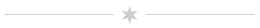# electrodynamicsTips for Assignment 3

In class we discussed the setup of various Coulomb integrals that show up on homework 3. What about problems 5 and 6, involving Gauss's Law? Here are a few tips to help if you are stuck.

On problem 5 the charge distribution is spherically symmetric because you can completely describe the charge at any point using only its distance from the center of the sphere. So the electric field has the form $$E(r)\,\hat{r{}}$$ like the example from class, and your Gaussian surfaces should be spheres with variable radius $$r$$. There are three cases to consider for the radius of the Gaussian surface: $$0 \leq r < R_{i}$$, $$R_{i} < r < R_{o}$$, and $$r > R_{o}$$. When determining the enclosed charge you will need to integrate over the volume inside your Gaussian surface. I will refer to points inside the surface with coordinates $$r'$$, $$\theta'$$, and $$\phi'$$. Then $$d\tau' = (r')^2 \sin \theta' dr' d\theta' d\phi'$$ and $q_{enc} = \oint_{GS} \!\!\!d\tau' \rho(\vec{r{}}') = \int_{0}^{2\pi} \!\!\! d\phi' \int_{0}^{\pi} \!\!\!d\theta' \sin \theta' \int_{0}^{r} \!\!\!dr' (r')^2 \rho(r')$ Be careful! Depending on the value of $$r$$, you might have to break the integral over $$r'$$ into two or more parts. For instance, if $$R_{i} < r < R_{o}$$ then $$\rho(r') = 0$$ for $$0 \leq r' < R_i$$ and $$\rho(r') = k / (r')^2$$ for $$R_{i} < r^{\prime} < r$$

On problem 6 the charge distribution has planar symmetry. We just need to know the distance $$z$$ from a point to the $$x$$-$$y$$ plane to describe the charge. If $$|z| < d$$ then there is a constant volume charge density at that point, and if $$|z| > d$$ then there is no charge at that point. We can conclude that the electric field has the form $$E(z) \hat{z{}}$$. For your Gaussian surface consider a rectangular box with its bottom at $$z_1$$, its top at $$z_2$$, and an area $$A$$ on the top and bottom faces. (Why don't the areas of the other four faces matter here?) Consider the the flux and enclosed charge for the cases $$z_{1} < z_{2} < d$$, $$z_1 < d < z_2$$, and $$d < z_1 < z_2$$. (Remember that the normal vector for a closed surface points from inside to outside, so in this case it is $$+\hat{z{}}$$ on the top of the Gaussian surface and $$-\hat{z{}}$$ on the bottom.) You probably assumed $$0 < z_1 < z_2$$ as you did this; what should the field look like for negative values of $$z$$? That is, based on the symmetry here, what do you expect for the field at the point $$(0,0,-z)$$ compared to $$(0,0,z)$$? Does this help you narrow down anything about the field that wasn't clear from the three cases above?

Back to the main page